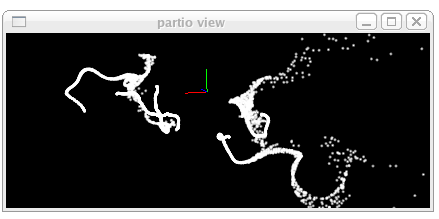## Usage Examples

### Tools

One handy thing that the Partio provides is simple command line tools to manipulate and example particle data. For example you can get information on a particle file of any type by using "partinfo".

```\$ partinfo test.bgeo
Number of particles:  9998

Type      Count     Name
----      -----     ----
VECTOR    3         position
VECTOR    3         v
INT       1         id
INT       1         parent
FLOAT     3         Cd

particle 0 position 0.536567 1.17344 0.523275
particle 1 position 0.495414 0.913424 0.434151
```
You can also view particles in a simple standalone (and very quick to load) particle viewer
```\$ partview test.bgeo
```Particles can easily be converted from one format to another such as from bgeo to RenderMan PTC.
```\$ partconv test.bgeo test.ptc
```

## C++ API

In C++ particle files can be created and added in a similar way. We have an iterator that allows quick iteration through data in the same way regardless of the internal format. As an example of computing the average position of all particles in a set.

```  // open file
if(!simple) die("failed to open test.pdb");

// prepare iteration
auto iterator=simple->begin(), end=simple->end();
ParticleAttribute posAttr;
if(!simple->attributeInfo("position",attr) || attr.type != VECTOR || attr.count != 3)
die("failed to get position as vector of size 3");

ParticleAccessor posAcc;

// compute sum
float avg = {0,0,0};
int cnt=0;
for (auto it=simple->begin(); it!=simple->end(); ++it) {
float* data = posAcc.raw<float>(it);
for(int k=0; k < 3; k++) {
avg[k] += data[k];
}
cnt++;
}
for (int k=0; k < 3; k++) {
data[k] /= cnt;
}

simple->release();
```

## Python API

Often particles need to be manipulated after the fact to have additional or fewer attributes. To compute aggregate statistics on a file etc. The python API to partio allows you to do this very quickly. The following code increases the radii on particles by 2, if within a distance of one of the origin.

```#!/bin/env python
import partio

filenameIn,filenameOut=sys.argv[1:]
for i in p.numParticles():
if dist(p.get(pos)) <= 1:
partio.write(filenameOut,p)
```

PARTIO SOFTWARE